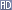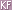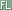Math Portal Home > 9 - 12

General | PreK - 2 | 3 - 5 | 6 - 8 | 9 - 12 | 13 - 20

9 - 12

 AAA Mathhttp://www.aaamath.com/index.html AAA Math illustrates and provide interactive arithmetic exercises and problems. They contain a series of basic math lessons which are a resource that can be used by math students in formal elementary education math classes, home schooling or elsewhere. These interactive lessons are much more conducive to learning math skills than traditional textbooks and work sheets. The Abacus http://www.ee.ryerson.ca:8080/~elf/abacus/intro.html The art of calculating with beads. Activities in Mathhttp://www.syvum.com/math/ Excercizes in Algebra, arithmetic, word problems, and Trigonometry. Algebra Helphttp://www.Algebrahelp.com Algebra Help uses some of the latest technology to help you learn and understand Algebra. This website features lessons to learn or refresh old skills, calculators that show you how to solve problems step-by-step, and interactive worksheets to test your skills. Algebra Review in 10 Lessons http://math.uakron.edu/~dpstory/mpt_home.html Algebra Review is a review of High school Algebra. Though written to prepare a student for the Math Placement Exam II at The University of Akron, the tutorial is suitable of all students. Algebra Solutionshttp://www.gomath.com/Algebra.html Enables students to get solutions to problems, practice Algebra skills, and find mini-lessons. Algebra Tutorial - Ms. Lindquist:The Tutorhttp://www.Algebratutor.org An intelligent tutoring system that teaches student how to translate Algebra word problems into Algebra expressions. Ask Dr. Math http://www.mathforum.org/dr.math There's always a doctor on call to attend to math emergencies at Ask Dr. Math. Email these doctors your questions and they'll respond with helpful hints and creative suggestions for solving math stumpers. Read answers already online, or submit new queries. Remember, the Math Doctors are in. Big Chalkhttp://www.bigchalk.com/cgi-bin/WebObjects/WOPortal.woa/db/Home.html Bigchalk harnesses the power of online content to create engaging learning environments that promote student achievement. Choose a subject and a grade level (elementary, middle, or "High school and beyond"), then drill down to get more specific, and you'll get links to pertinent, well-chosen Web sites for further research. You'll find better content much faster than if you were using a general-purpose search engine. BrainPOPhttp://www.brainpop.com/math/ Animated characters delve into the worlds of numbers and operations, measurements, Geometry, Algebra, and data analysis. Calculatorshttp://www.math.com/students/tools.html Useful calculators from basic, square root, scientific and Trigonometry. Calculator-in-the-URLhttp://x42.com/help_urlcalc Did you know you can add, subtract, multiply, divide, and do all sorts of other mathematical calculations right in the URL line of your browser? It's true, and the Calculator-in-the-URL will show you how it's done. Calculus for your Kids http://www.maa.org/pubs/calc_articles.html Mathematicians Ron Larson, Robert P. Hostetler, and Bruce H. Edwards have selected articles, magazines and journals on Calculus from Math Association of America periodicals to supplement their textbook, Calculus with Analytic Geometry, Seventh Edition. Calculus Help http://www.Calculus-help.com/ Calculus Help offers content for both advanced High school students and college Calculus students. In addition to free multimedia tutorials, it continues to offer a practice problem just about every week that is answered in plain, everyday English the following week. Computational Science Education http://csep1.phy.ornl.gov/csep.html CSEP is an electronic book for teaching Computational Science and Engineering. The intended audiences are students in science and engineering at the advanced undergraduate level and higher. Tutorials for freely available networking and visualization software are included and have attracted a range of users, including high-school students and people from the commercial sector. CoolMathhttp://www.coolmath.com Explore this amusement park of mathematics! Have fun with interactive activities and games like Lemonade Stand and Arithmattack. There's even stuff for parents and teachers too. Cool Toolshttp://www.math.com/students/tools.html Find all kinds of calculators and converters. Type in an equation and the applet will draw your graph on an x,y coordinate grid. You can graph, one, two or more equations and compare. Need to solve a quadratic equation, enter the coefficients and the quadratic equation will output the solution. Curious and Useful Mathhttp://personal.cfw.com/%7Eclayford/ Contains tricks and rules for working through certain types of math problems. Damping Functionshttp://www.coolmath.com/dampfunction1.htm These pages will explain damping functions and the very cool way they are used in music. Dau Math Refresher http://cne.gmu.edu/modules/dau/math/index.html This module covers fractions, exponents, logarithms, quadratic equations, series, functions, derivatives, and integrals. Dave's Short Trig Course http://aleph0.clarku.edu/%7Edjoyce/java/trig/ This site allows you to learn a bit about Trigonometry or brush up on what you may have previously learned. There are a few exercised for you to work on and the short answers are provided as well. Discovery School - WebMathhttp://school.discovery.com/homeworkhelp/webmath Unlike most math sites, WebMath is not a database of questions and answers. WebMath allows users to enter their problem, then WebMath searches it database for similar problems with will allow the student to see similar solutions to their problem. WebMath provides the frustrated student with a place to start when they are stumped by a math problem. Dositeyhttp://www.dositey.com Dositey.com offers a dynamic collection of interactive educational modules and printable worksheets for grades K-8. Free interactive lessons, exercises and worksheets, educational games, open-ended questions in Math and Language Arts, and more. Lessons, exercises, games, and other learning activities on the site are specially designed to be fun and easy to use. e-Calculus http://www.math.uakron.edu/~dpstory/e-Calculus.html e-Calculus is a Calculus I tutorial. Features include verbose discussion of topics, inline examples and exercises with solutions, multiple choice quizzes, and pop-up graphics. Egyptian Mathematics http://www.eyelid.co.uk/numbers.htm Learn how to read and write the seven different symbols the Egyptians used to solve math problems. Eric Weisstein's World of Mathematics http://mathforum.org/library/more_info.html?id=2832 A full-text searchable, comprehensive alphabetical encyclopedia of math terms, equations, and derivations, with explanations, examples, references, and much more. Euclid's Elements http://aleph0.clarku.edu/%7Edjoyce/java/elements/toc.html An online guide to Geometry. Explore Mathhttp://exploremath.com If the mere thought of Algebra or Calculus makes you (or your kids) break out in hives, try a new approach to math: Play with the interactive tools at ExploreMath.com. Select a category (Conic Sections, Quadratics, Complex Numbers), and then a problem or tool to experiment with. The big advantage here is that animated equations show you visually how changing variables will alter the curve of an arc or the slope of a line. The Explorer http://unite.ukans.edu/explorer-dB/browse/static/Mathematics/index.html The Explorer is collection of educational resources, instructional software, lab activities, lesson plans, and student created materials for K-12 mathematics and science education. e-zGeometryhttp://www.e-zGeometry.com/ The e-zGeometry web site supports Geometry students by recreating the classroom experience again but this time in web format. The e-zlibrary contains an interactive Geometry textbook, a glossary of geometrical terms, teacher/student classroom video examples, and many challenging investigations through java applets. Famous Problems in the History of Mathematics http://mathforum.org/isaac/mathhist.html The purpose of this site is to present a small portion of the history of mathematics through an investigation of some of the great problems that have inspired mathematicians throughout the ages. Included are problems that are suitable for Middle school and High school math students, with links to solutions, as well as links to mathematicians' biographies and other math history sites. FCAT Explorerhttp://www.fcatexplorer.com FCAT Explorer offers students fun, effective practice on skills outlined in the Sunshine State Standards and skills tested on the FCAT. FCAT Explorer also enables teachers to create reports and monitor student performances and progress. Students enrolled in FCAT Explorer's online programs may use many of its' interactive programs at home, as well as in school and may be monitored by parents. Frank Potter's Science Gymshttp://www.sciencegems.com/math.html Collection of mathematics links arranged by grade level. The Fruit Gamehttp://www.2020tech.com/fruit/index.html On the table before you sit several piles of fruit. Players take turns removing fruit from the table. On your turn, you must pick one kind of fruit and remove at least one of them from the table. The object of the game is to remove the last fruit from the table. Fun Mathematics lessons http://math.rice.edu/~lanius/Lessons/ Test your math skills with these fun problems based on fractions and Geometry. Geometry Lesson Plans http://mathforum.org/Geometry/geom.units.html This list contains some of the best Geometry lesson plans or materials on which to base them. Graphics for the Calculus Classroomhttp://www.ima.umn.edu/~arnold/graphics.html Graphics that illustrate the main principals of Calculus. Graphing and Plotting http://www.math.com/students/graphing.html Full-featured instant graphing solution, no Java needed. Generate a plot of almost any function or relation. The advance plot allows you to graph multiple equations on the same graph. You can print graphs too! Graphing Vector Calculator: an Interactive Java Applet http://www.frontiernet.net/~imaging/vector_calculator.html Constructed for K-12 math students, this site allows you to learn about Vectors and Vector Operations by sketching and playing with them. Drag your mouse across the grid to create the vectors and then follow the directions to perform calculations on them. Harcourt Multimedia Math Glossarywww.hbschool.com/glossary/math This interactive math glossary provides a searchable database of math terms by grade level. Students in grades one through eight can search the glossary for math definitions accompanied by illustrations and, where appropriate, animated examples. High School Student Center http://mathforum.org/students/high Students, parents and educators will find links to math resources, including software, online Internet activities, brain teasers, puzzles and a link to the Internet Mathematics Library. The high school math search link will provide information to other math sites on the High school level. The High School Students Guide to Programming http://members.fullnet.net/jbowen Dedicated to the premise that one of the defining characteristics of mathematics education during the early part of the 21st century will be the full integration of programming throughout the high school mathematics curriculum as an accepted and fundamental problem solving technique. HMC Mathematics Online Tutorial http://www.math.hmc.edu/Calculus/tutorials/ An online tutorial centered on Calculus. Illuminations http://illuminations.nctm.org/index2.html Internet resources to improve the teaching and learning of mathematics for all students. Provide professional development for teachers of mathematics and provide Standards-based resources for the classroom use. Intel® Innovation Education Webhttp://www97.intel.com/education/index.asp "Intel® Innovation in Education" provides resources supporting the use of technology in classrooms to improve student learning. It also provides information about Intel's global programs to help improve math, science, and technology education Interactive Math Tutorhttp://www.interactivemathtutor.com InteractiveMathTutor.com provides math tutoring services to all ages of individuals in all levels of math. Interactive Tutor strives to provide tutoring services customized to the needs of the individual students. Students may choose online tutoring, homework assistance, worksheets, telephone and e-mail assistance for all mathematical subjects from early education through adult education. Internet Mathematics Library http://mathforum.org/library/ The Math Forum's Internet Mathematics Library contains a Recreations category, with topics from the lighter side of mathematics. The Library also offers collections of math games and math-oriented sports sites. An Introduction to Trigonometry http://www.ping.be/%7Eping1339/gonio.htm Moving figures which help students to grasp the meaning of Trigonometry concepts. The MacTutor History of Mathematics http://www-groups.dcs.st-and.ac.uk/~history A collection of interesting facts about math history, including biographies of famous mathematicians. Manipula Math Applet Collections: Trigonometry http://www.ies.co.jp/math/products/trig/menu.html Interactive programs that can be manipulated and animations that help to grasp the meaning of mathematical concepts. The Math Archives http://archives.math.utk.edu/Calculus/crol.html The Calculus Resources online area of the Mathematics Archives contains information and links to numerous Internet resources, which could be used for teaching and learning of Calculus. Math Central http://mathcentral.uregina.ca/index.html Math Central is a service for people involved in mathematics education from kindergarten to grade twelve. Math Central provides a meeting place for teachers to share resources, a service to teachers, students and parents who may need an answer to a mathematical question and a facility to allow teachers to share information with other teachers. Math.com - The World of Math Onlinehttp://www.math.com Math.com is dedicated to providing revolutionary ways for students, parents, teachers, and everyone to learn math. Math.com offers homework help, practice programs, games and online calculators and other tools. Math Counts - Problem Solving Strategies mathcounts.org/Problems/strategies.html These problem solving strategies from MATHCOUNTS can help you solve any math problem, no matter how complicated it may be! Try them the next time you are stuck on a math question. Math Drills http://www.saab.org/mathdrills/ Problems are randomly generated, scores are kept, details and hints are available. Math Quotations http://math.furman.edu/~mwoodard/mquot.html Hundreds of Quotations by famous Mathematicians. Math Reference Tableshttp://www.math.com/tables/tables.htm This site provides instant access to dozen of formulas, tables and properties. MathWorldhttp://mathworld.wolfram.com/ MathWorld is a comprehensive and interactive mathematics encyclopedia intended for students, educators, math enthusiasts, and researchers. Although it is often difficult to find explanations for technical subjects that are both clear and accessible, this website bridges the gap by placing an interlinked framework of mathematical exposition and illustrative examples at the fingertips of every internet user. Mathematics WWW Virtual Library http://www.math.fsu.edu/Virtual/ This collection of Mathematics-related resources is maintained by the Florida State University Department of Mathematics as a free service to the online community. New York Times Daily Lesson Planhttp://www.nytimes.com/learning/teachers/lessons/mathematics.html The New York Times Daily Lesson Plans allows teachers to search or browse their collection of lesson plans which will help them integrate the news in to the curriculum. Mathematics lesson are generated for middle and High school students. Teachers can also sign up to have lesson plans delivered directly by e-mail. online Conversionhttp://www.onlineconversion.com Find calculators that will perform conversions between almost every measurement type imaginable, from science to cooking to math. Plane Math http://www.planemath.comWelcome to PlaneMath, a place to learn cool things about math and aeronautics. The student activity pages will allow students to go directly to lessons, which relate to various aeronautics skills. Although the stated mission of this project is to stimulate and motivate students with physical disabilities in grades 4-7 to pursue aeronautics-related careers via the development and delivery of accessible math education materials on the Internet, the site can be viewed by all students interested in learning about math and aeronautics. Practical Algebra Lessonhttp://www.purplemath.com/modules/modules.htm Practical Algebra lessons that contain tips, hints and examples, and point out common mistakes. Prime Links++ http://primes.utm.edu/links/ Here you can find links to all sorts of information about prime numbers and their uses. Have a number and not sure it's a prime, plug it in and find out. Need to know the first 1000 prime numbers? You find this information and much more at this site! The Prime Pages http://primes.utm.edu/ Everything you ever wanted to know about prime numbers, including their history, the largest known prime, how to find primes, how many primes there are, and special types of primes. Probability Tutorials http://www.probability.net/ Probability.net offers tutorials in measure theory, lebesgue integration and probability. Work through these topics by solving a series of exercises (solutions provided). Quick Mathhttp://www.quickmath.com QuickMath is an automated service for answering common math problems over the internet. Think of it as an online calculator that solves equations and does all sorts of Algebra and Calculus problems - instantly and automatically! QuickMath will automatically answer the most common problems in Algebra, equations and Calculus faced by high-school and college students. Roman Numeral Conversionhttp://www.ivtechstudios.com/roman/ Enter the number you want to convert (Arabic or Roman) and instantly see the equivalent! S.O.S. Mathematicswww.sosmath.com Browse free math review materials in topics ranging from Algebra to differential equations on SOS Mathematics. The 2,500 pages with clear, concise explanations are geared directly to High school and college students. The Stock Market Game http://www.smg2000.org/ This is an "electronic simulation of Wall Street trading" where students in grades 4-12 form teams and invest \$100,000. Tracking, buying, and selling, they learn about the stock market and related functions. Established in 1977 and now updated for Internet use, SMG offers teacher training in addition to the onsite glossary and FAQ. Superkids Math Worksheet Creatorhttp://www.superkids.com/aweb/tools/math/index.shtml Have you ever wondered where to find math drill worksheets? Make your own here at SuperKids for free! Simply select the type of problem, the maximum and minimum numbers to be used in the problems, then click on the button! A worksheet will be created to your specifications, ready to be printed for use. Syvumhttp://www.syvum.com/math/Trigonometry.html Online Trigonometric definitions and functions. Trigonometry Realms http://id.mind.net/%7Ezona/mmts/TrigonometryRealms/TrigonometryRealms.html Animated designs are used to explain Trigonometry. Visual Calculus http://archives.math.utk.edu/visual.Calculus/ Visual Calculus offers a collection of modules that can be used in the studying or teaching of Calculus. Webtrig http://www.math.uakron.edu/~tprice/Trig/ Webtrig is a World Wide Web tutorial covering the basics of Trigonometry. The lessons include an introduction to angles and their measurement, definitions of the trigonometric functions, gaphs of trigonometric waves, an introduction to trigonometric identities, and techniques for solving trigonometric equations. Top

 Math Portal Home > 9 - 12